# Activation functions: Why are they important and how to use them?Source

# Activation Functions:

When you build a neural network, one of the decisions you can make is the choice of an activation function. Activation functions give neural networks the power of mapping nonlinear functions. Imparting non-linearity to the neural network helps it to solve complex problems. They help your model to capture squiggly looking patterns that you will often encounter in real-life data. This is essential since nature in general doesn’t always operate linearly.

Simply put, the activation function squishes the output of the summation operator into a different range of values that represent how much a node should contribute.

# What should we look for in an activation function?

• Don’t vanish: A very serious issue when it comes to activation functions is sometimes their gradients become so small during training that they seemingly vanish. This is known as the vanishing gradient problem. When that happens, over time as the gradients get smaller, the weight update is negligibly small. That means that at some point the model stops learning. We don’t want that to happen.
• Zero-centered: When the output of the hidden layers are zero centered the data is normally distributed. If the data is not normally distributed, it takes more computation time for convergence.
• Computational efficiency: Usually neural networks are deep and take a lot of time to compute, so computational efficiency is an important factor in the choice of the activation function.
• Differentiable: We optimize and train neural networks using the backpropagation algorithm, so the activation function must be differentiable.

# Types of Activation function:

1. Sigmoid: Sigmoid is a very well known activation function. It’s a nonlinear function so it helps the model capture complex patterns.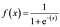Source

The range of a sigmoid function is between 0 to 1. That means that the function is not zero centered. The activation function is also notorious for causing the vanishing gradient problem when used in hidden layers. Take a look at the graph below.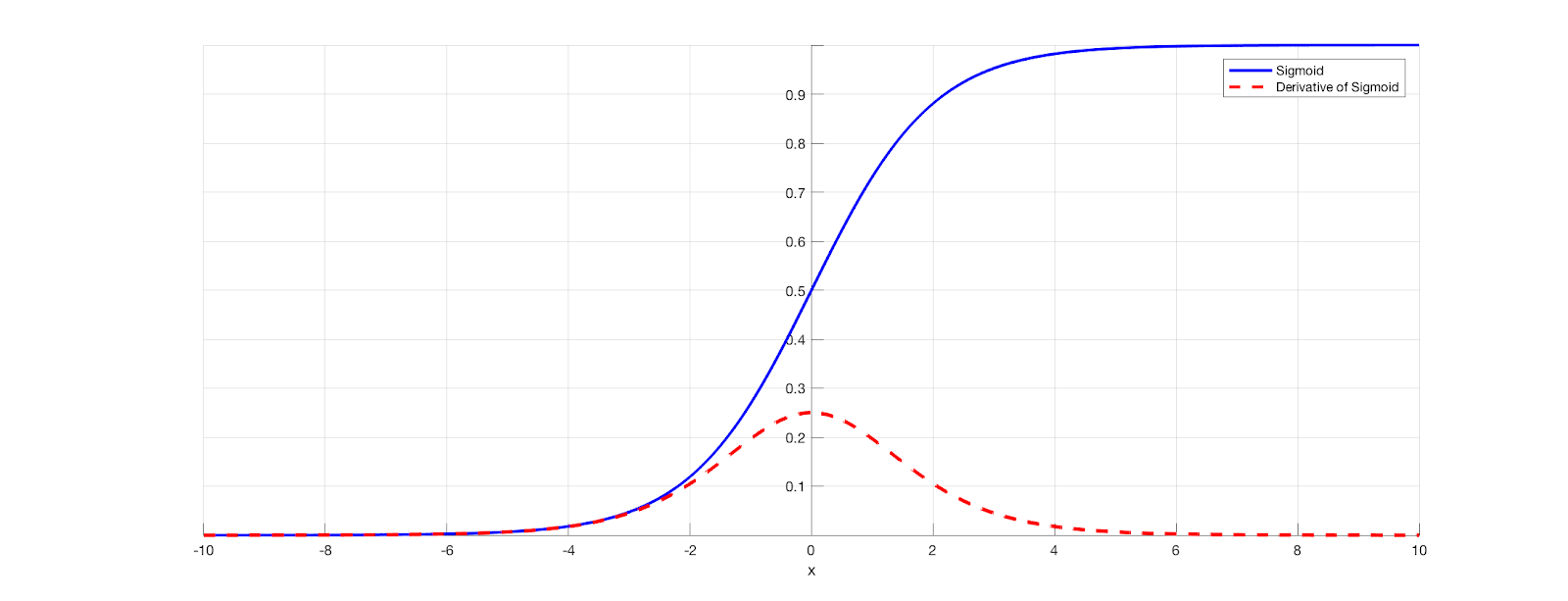Source

Notice how that gradient is tiny as the value increases in the positive direction and decreases in the negative? That’s going to cause the problem. So it’s wise to use the sigmoid as an activation function in the output layer of a binary classifier. So the output will give a value between 0 and 1 that represents the probability of an observation belonging to a class.

2. Softmax: We know we can use sigmoid for binary classification, but what if I had more than one class? What if I wanted to classify across multiple classes? Sure, we could assign n nodes for ‘n’ classes with a sigmoid activated value, ranging from 0 to 1. The only problem with that is the sum of n nodes will not necessarily equal 1. In other words, each node predicts that chance of belonging to a class, irrespective of other classes. I would prefer my activation function to take into account the probability of the observation belonging to other classes and giving me an overall chance of belonging to a class. I can do this with softmax.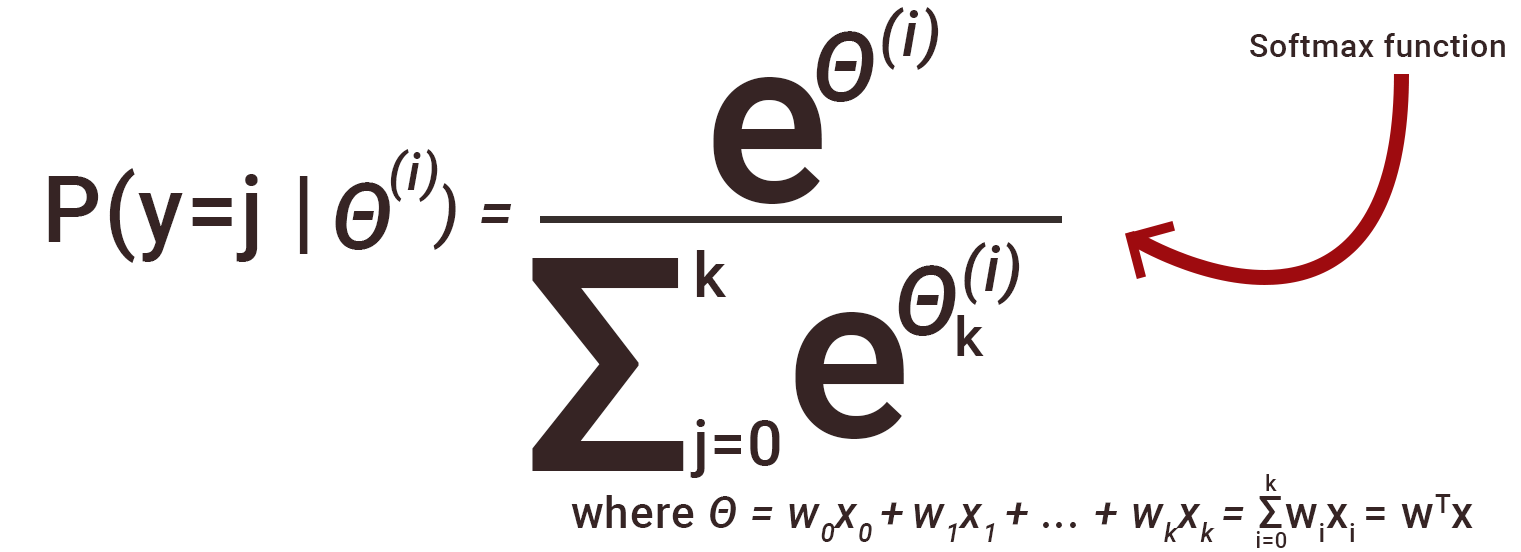Source

This will give me a value that measures the probability of belonging to a class against other classes. And so the output of the n nodes will add up to one.

3. Tanh: This activation function is similar to sigmoid and has a zero-centered property with output values ranging from -1 to 1. That makes tanh a more favorable option when compared to sigmoid. However, take a look at the derivative graph. The derivative has extremely small values for higher positive and negative values which bring us back to the vanishing gradient problem.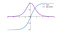Source

4. ReLU: Rectified Linear Unit is one of the most popular and widely used activation functions of all time. This activation function is preferred a lot for deep neural networks because it’s easy to train and is known to perform well.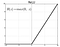Source

ReLu has been very successful when used with neural networks. One of the reasons why ReLu is so successful is that it is a non-saturating activation function. What that means is that the function doesn’t squeeze the input it gets from the linear function. It simply computes max(0, x) where x is an output of the affine function. Since ReLu assigns zero for negative values, it also acts as a feature selector. This operation is not computationally expensive so it makes our network lighter and it converges a lot faster. ReLu is bounded below and unbounded above. Bounded below means that as x approaches negative infinity, y approaches a constant ( 0 for x <= 0), and unbounded above means that as x approaches positive infinity, y also approaches positive infinity. Unboundedness above is good for faster training and bounded below acts as a regularizer by neglecting inputs that approach zero to negative infinity.

In case you’re wondering how ReLu is non-linear, it is a piecewise linear function that is defined to be 0 for all negative values of x and x for all non-zero values. Even though the reasoning is correct, it still isn’t a strictly non-linear function like sigmoid or tanh. So how does ReLu help capture complex patterns in data?

I built two simple neural networks to capture the pattern is an annulus data that I randomly generated using sklearn’s datasets. One used the ReLu activation function and the other used sigmoid.

Notice how ReLu creates a square-shaped decision boundary while sigmoid has a smoother edge? ReLu is capable of tilting a linear function to an angle. ReLu works well only when there is enough of it to create the decision boundary that generalizes well.

When there are enough neurons and layers, we can aggregate the ReLu functions to approximate any function just as well as other activation functions.

If you look at the derivative of ReLu, it is zero for negative values and 1 for positive values. Since ReLu assigns zero for negative values, these neurons never get activated leading to dead ReLus in our network.

5. Leaky ReLu: Leaky ReLu is a variant of ReLu designed to ensure that all neurons get activated. Instead of assigning zero to negative values, Leaky ReLu assigns a very small linear negative value. It introduces a slope value of 0.01 for negative values and computes f = max(0.01*x, x). Since the negative value now has a non-zero value, the derivative will also have a non-zero value. We will no longer have dead neurons. You can also use the parameterized ReLU function which allows you to specify ‘a’ to dictate the slope of the negative part. The only problem with leaky ReLu is vanishing gradients. Since it assigns a very low value to negative numbers, in deeper networks the gradients eventually vanish during backpropagation and the network fails to learn.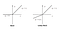Source

6. Parameterised Relu: This type of Relu is also designed to solve the dying relu problem. Parameterised Relu computes the function that is defined to be an*x for all negative values of x and x for all non-zero values, where a is a learnable hyperparameter.

• When a = 0, Parameterised Relu takes on the classic ReLu form.
• When a = 0.01, It becomes Leaky ReLu

7. Exponential Linear Unit (ELU): Take a look at the graph of ReLu and Leaky ReLu again, you will notice that both are not differentiable at zero. Elu is an adaptation of ReLu which forms a smooth curve at zero making it differentiable at all points. This property allows elu to converge to a loss of zero much faster than other variants of ReLu. Also, it solves the dying ReLu problem so it’s a win-win. On the con side, elu computes f = a(e^x — 1) for each x value which takes a hit computationally.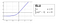Source

8. Swish: Swish is an activation function proposed by Google Brain Team in the year 2017. This activation function is very exciting because it beat the long-standing champion of activation function ReLu in terms of performance. Here’s the formula of Swish: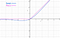Source

Swish also shares the bounded below and unbounded above property with ReLu, but it is differentiable throughout unlike ReLu. It is a non-monotonic function, the function is increasing and decreasing at different intervals of its domain. Swish also forms a smooth curve. This smoothness of swish helps the optimization process and it generalizes better.

# Swish Vs Relu:

On replacing Relu layers with Swish on the MNIST data set, researchers found that Swish performed much better.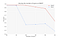Source: Swish paper

# When to use which activation function?

We’ve discussed popular activation functions, how do we decide what to use? Unfortunately, there is no one solid answer but there and tricks and techniques you can use. I am going to list some of them for you.

• Use sigmoid for output layer only for classification problems. Use softmax if you have more than 2 classes.
• Start with ReLu for the hidden layer activation function. If you encounter a dead neurons problem (dying ReLu), switch to LeakyRelu.
• The rule of thumb is to start ReLu and try out other activation functions.
• If you are using a non-zero centered activation function, use batchnorm to normalize between layers.

I’m changing.